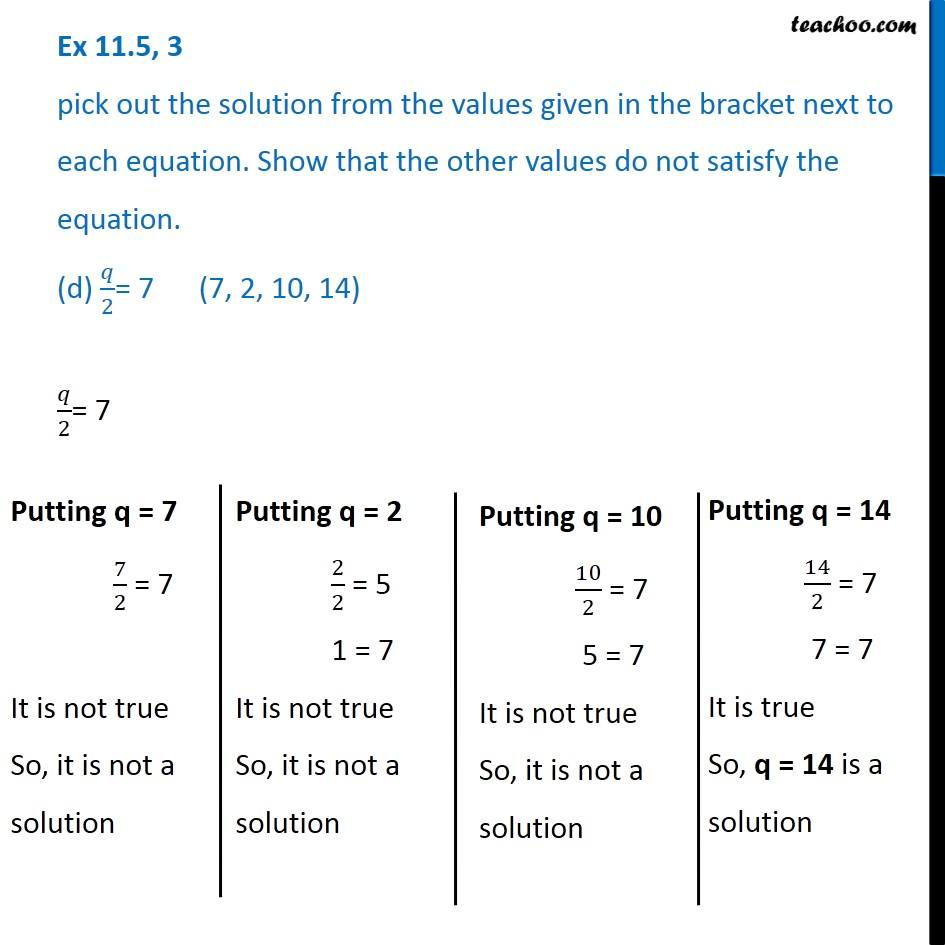Finding solutions of Algebra Equations - WorksheetLearn in your speed, with individual attention - Teachoo Maths 1-on-1 Class

### Transcript

Question 3 pick out the solution from the values given in the bracket next to each equation. Show that the other values do not satisfy the equation. (d) 𝑞/2= 7 (7, 2, 10, 14) 𝑞/2= 7 Putting q = 7 7/2 = 7 It is not true So, it is not a solution Putting q = 2 2/2 = 5 1 = 7 It is not true So, it is not a solution Putting q = 2 2/2 = 5 1 = 7 It is not true So, it is not a solution Putting q = 10 10/2 = 7 5 = 7 It is not true So, it is not a solution Putting q = 10 10/2 = 7 5 = 7 It is not true So, it is not a solution Putting q = 14 14/2 = 7 7 = 7 It is true So, q = 14 is a solution